# 字节面试题太坑了，刚回答完，就被面试官diss了！

### 题目

``````F(0) = 0,   F(1) = 1
F(N) = F(N - 1) + F(N - 2), 其中 N > 1

``````来源：力扣（LeetCode）

### 面试现场``````class Solution {
public int fib(int n) {
if(n == 0)
return 0;
if(n == 1)
return 1;
return fib(n - 1) + fib(n - 2);
}
}### 回到学校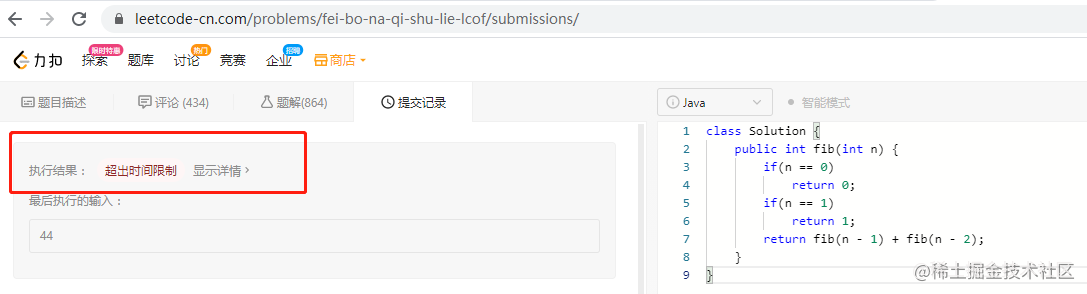### 记忆法递归``````class Solution {
public int fib(int n) {
int [] arr = new int[n + 1];
for (int i = 0; i < arr.length;i++) {
arr[i] = -1;
}
return fibWithArray(n, arr);
}
public int fibWithArray(int n,int [] arr) {
if (n < 2) {
return n;
}
if (arr[n] == -1) {
arr[n] = (fibWithArray(n-1, arr) + fibWithArray(n-2, arr)) % 1000000007;
}
return  arr[n];
}
}#### 记忆法递归例子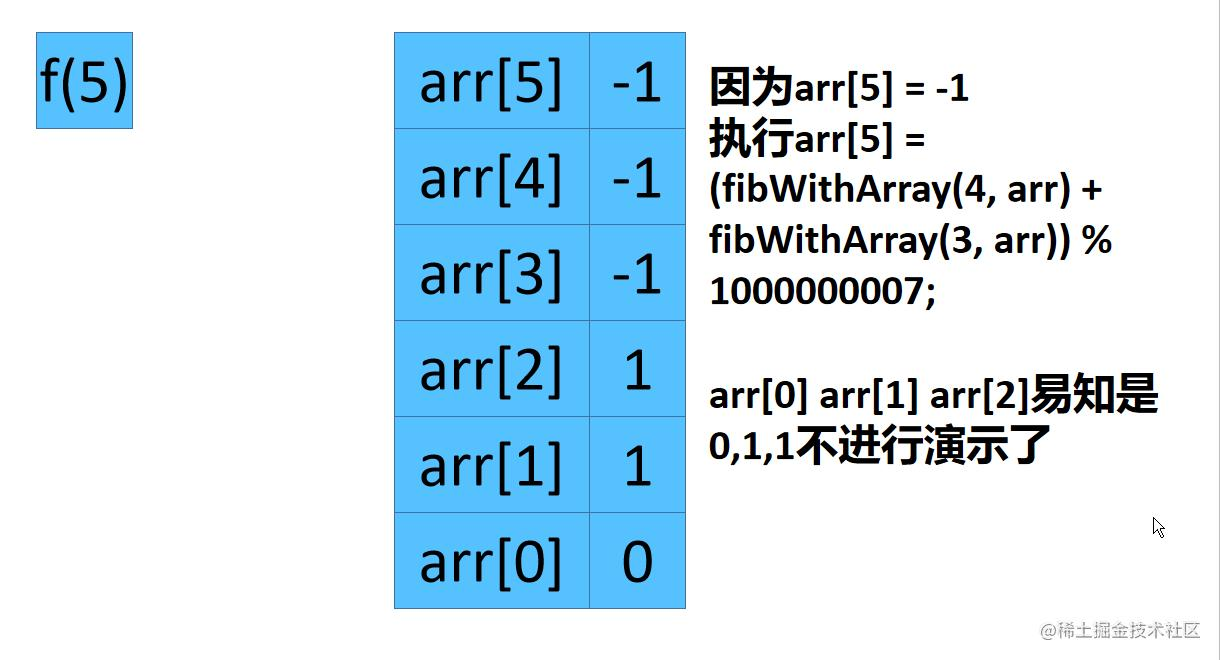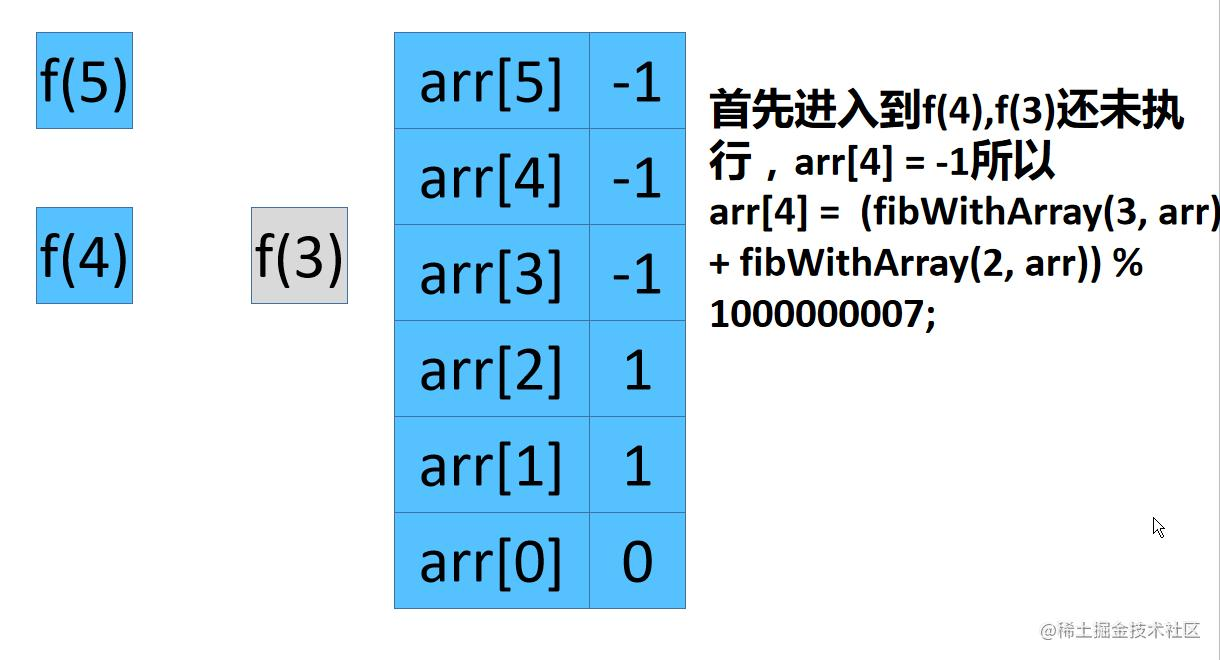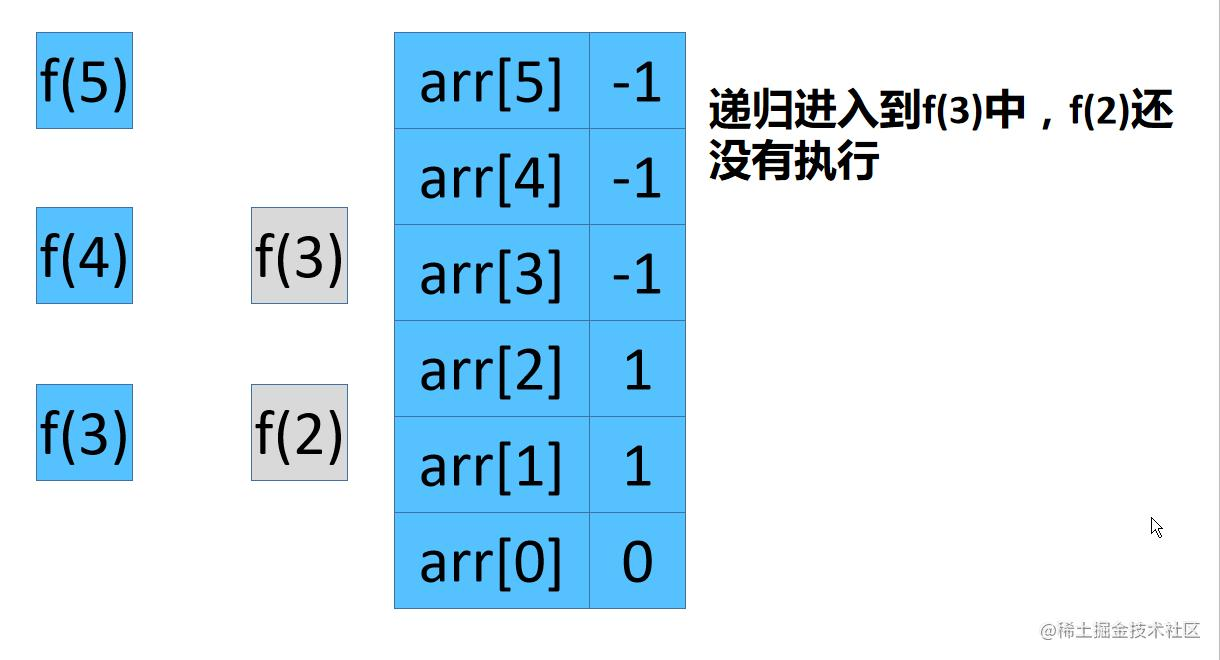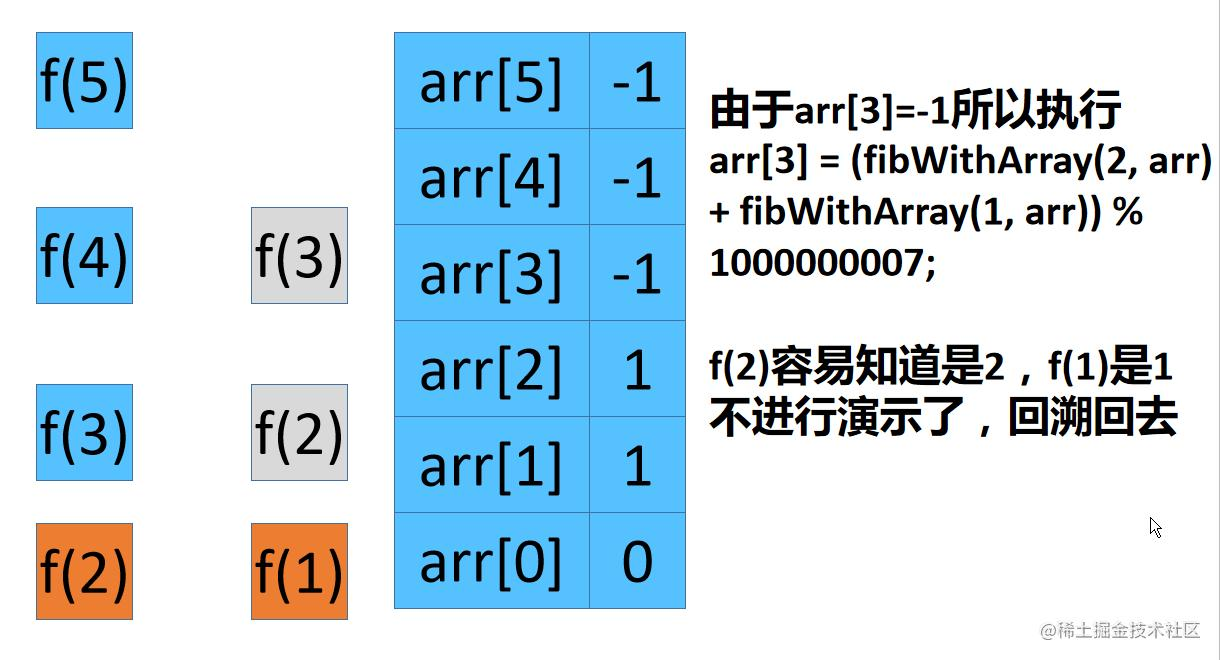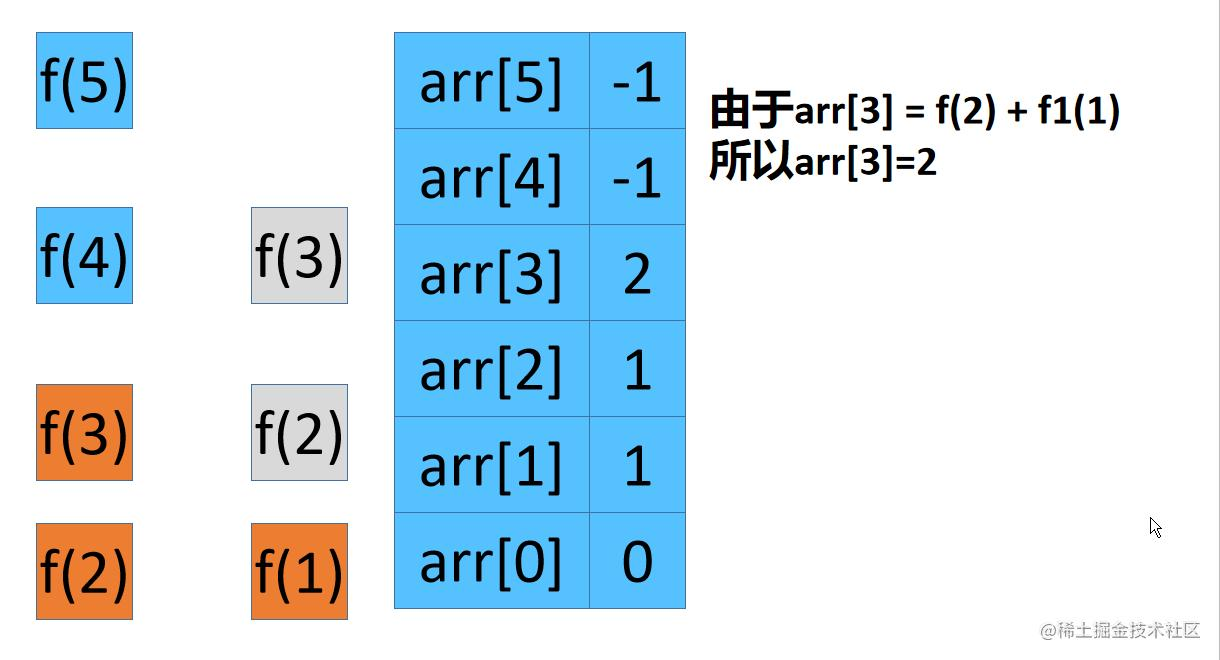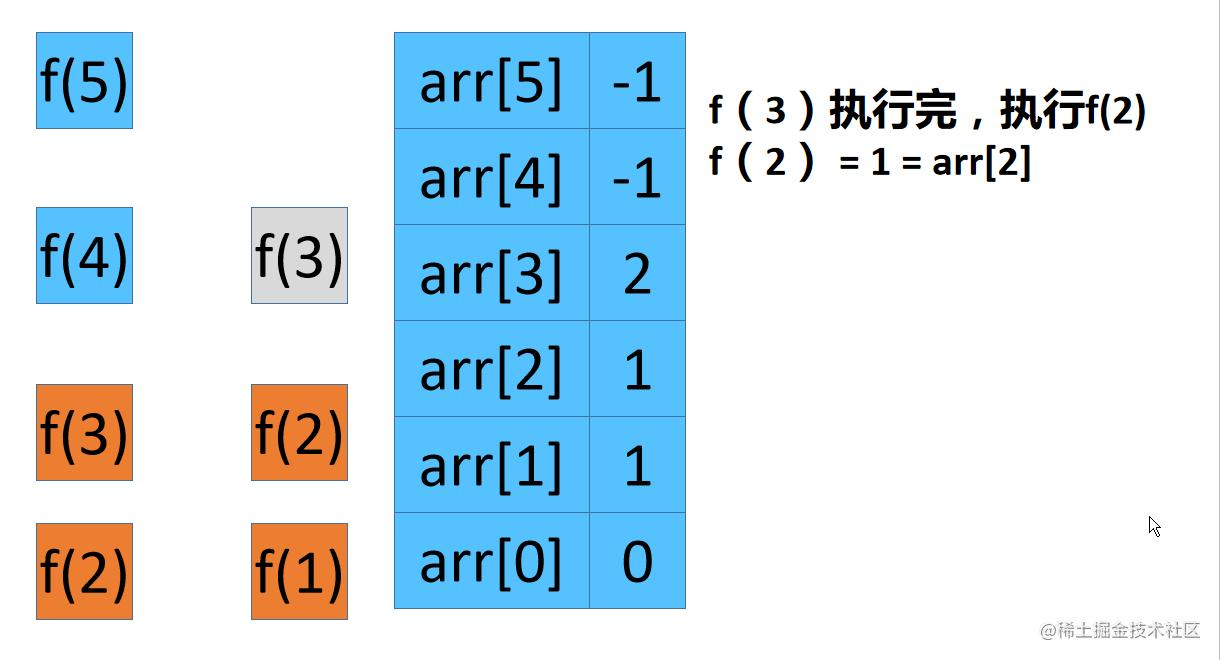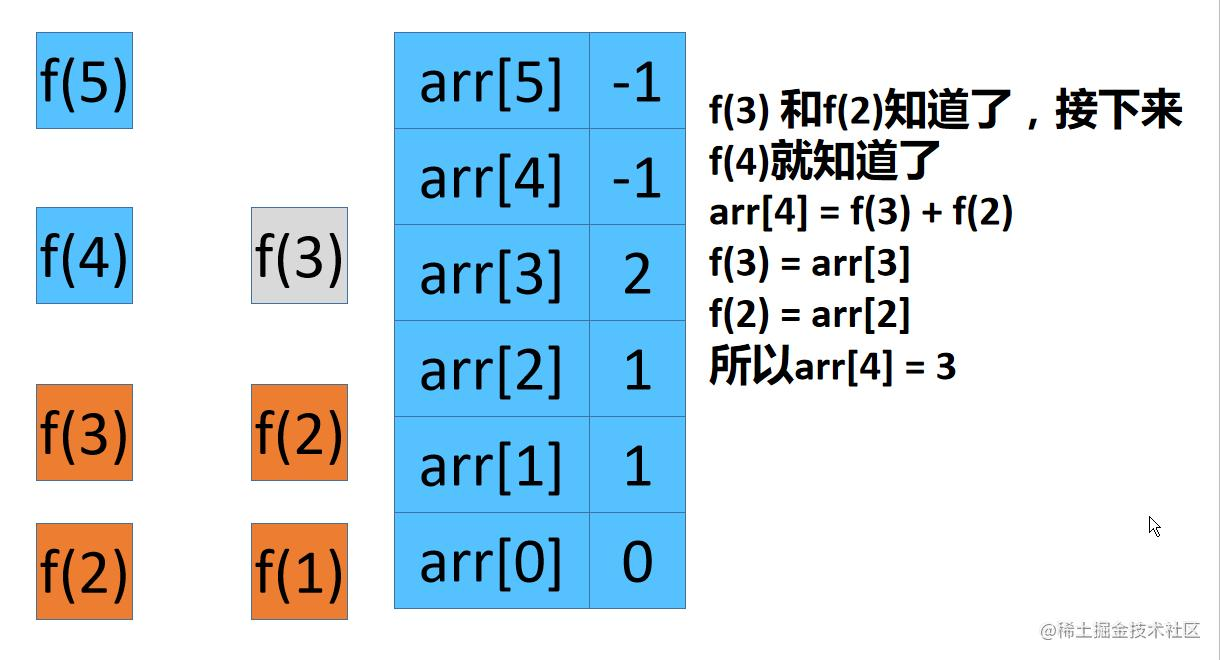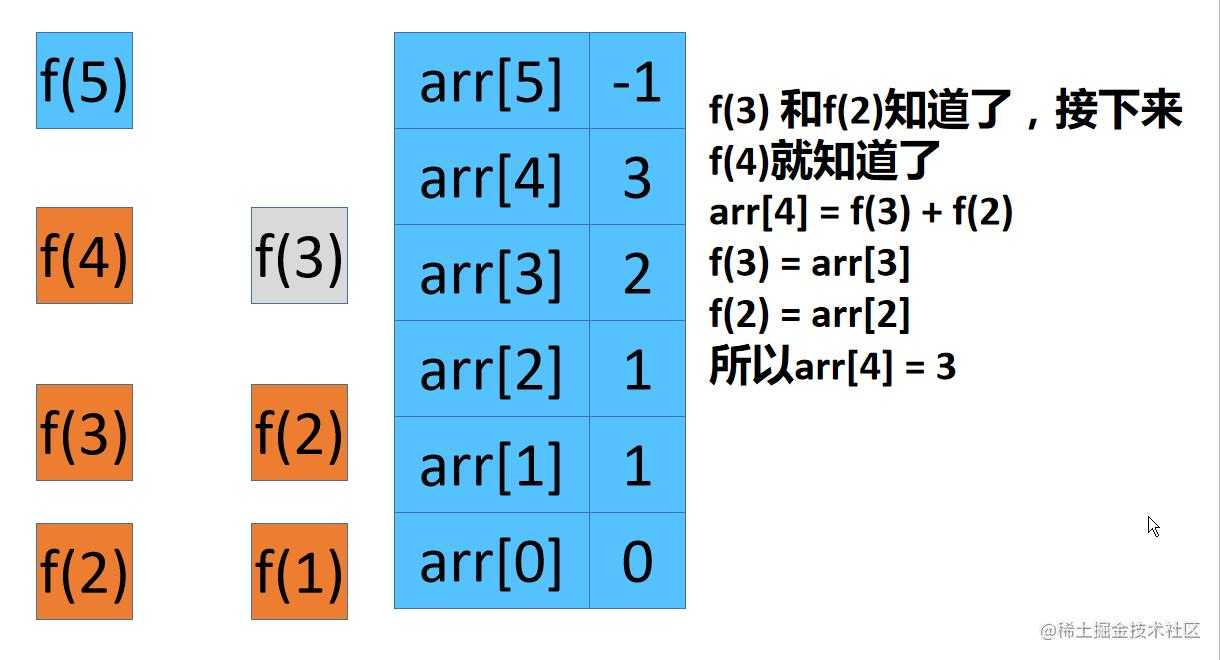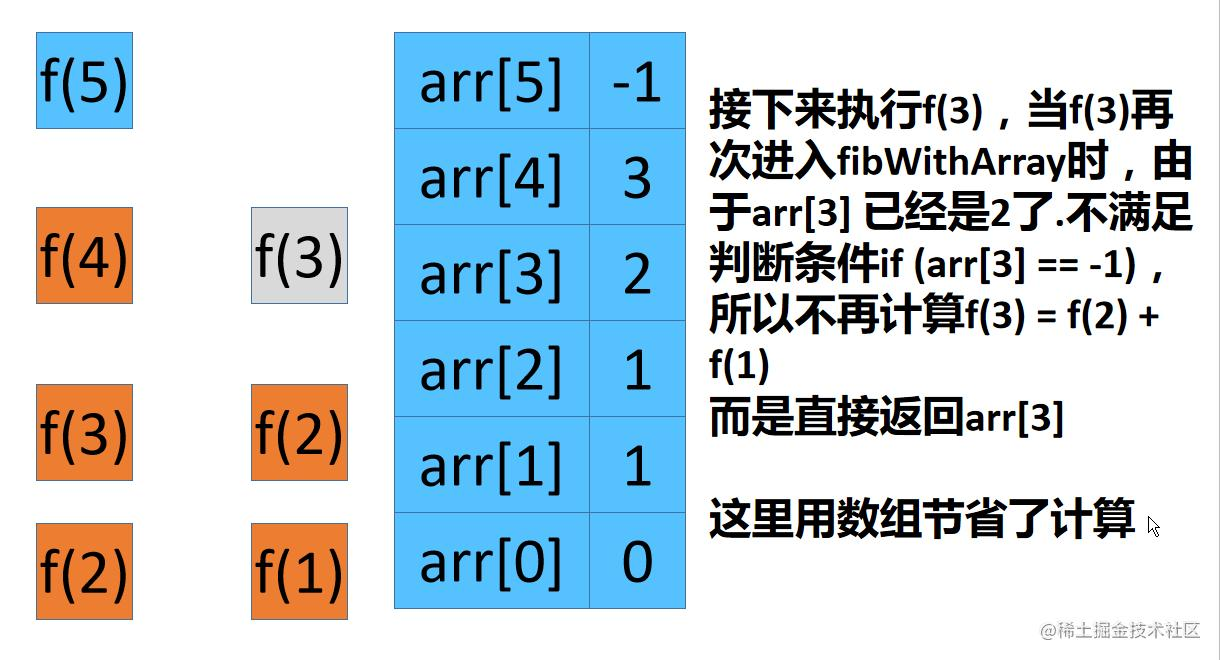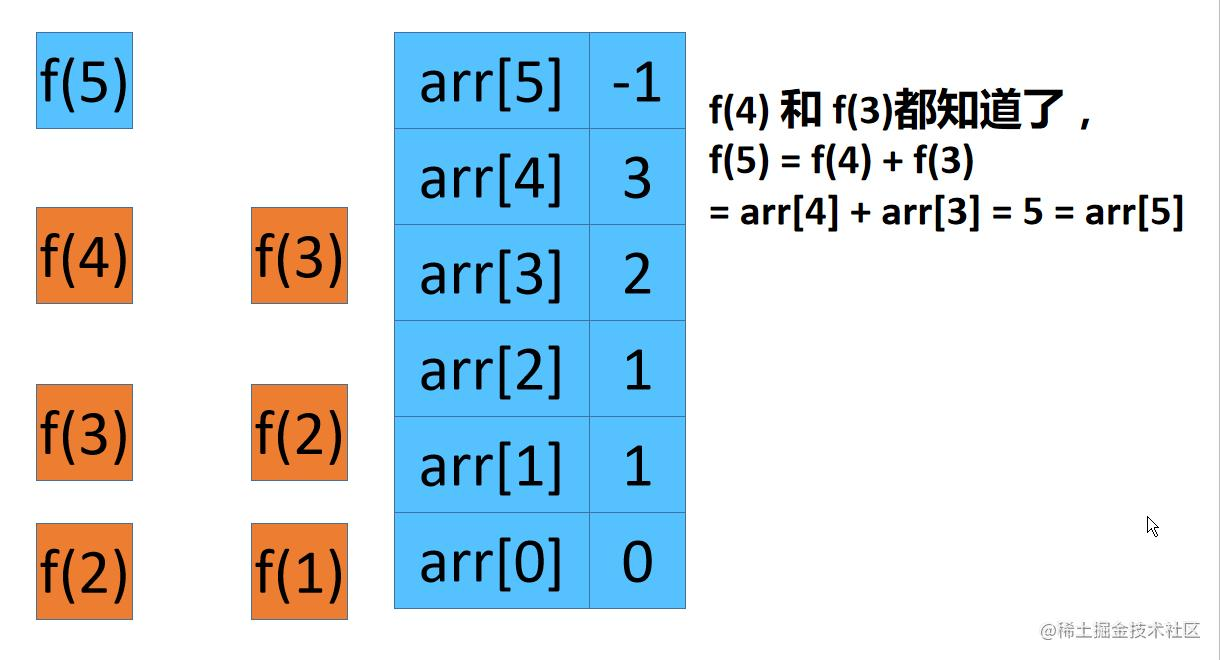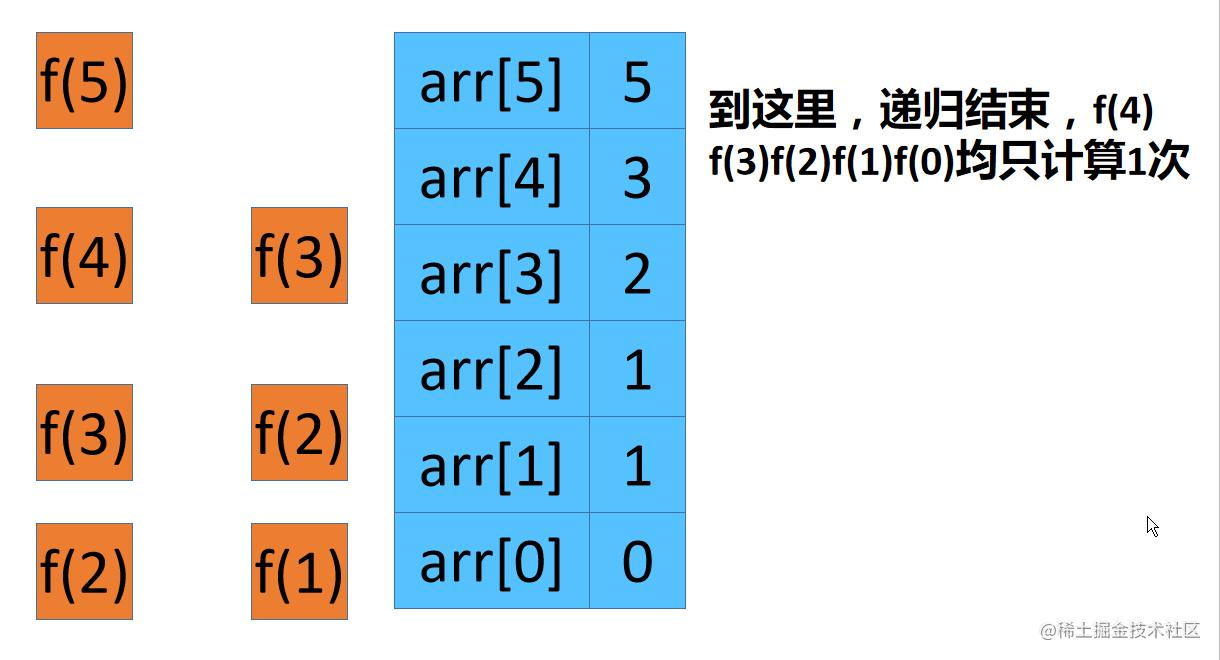#### 递归动画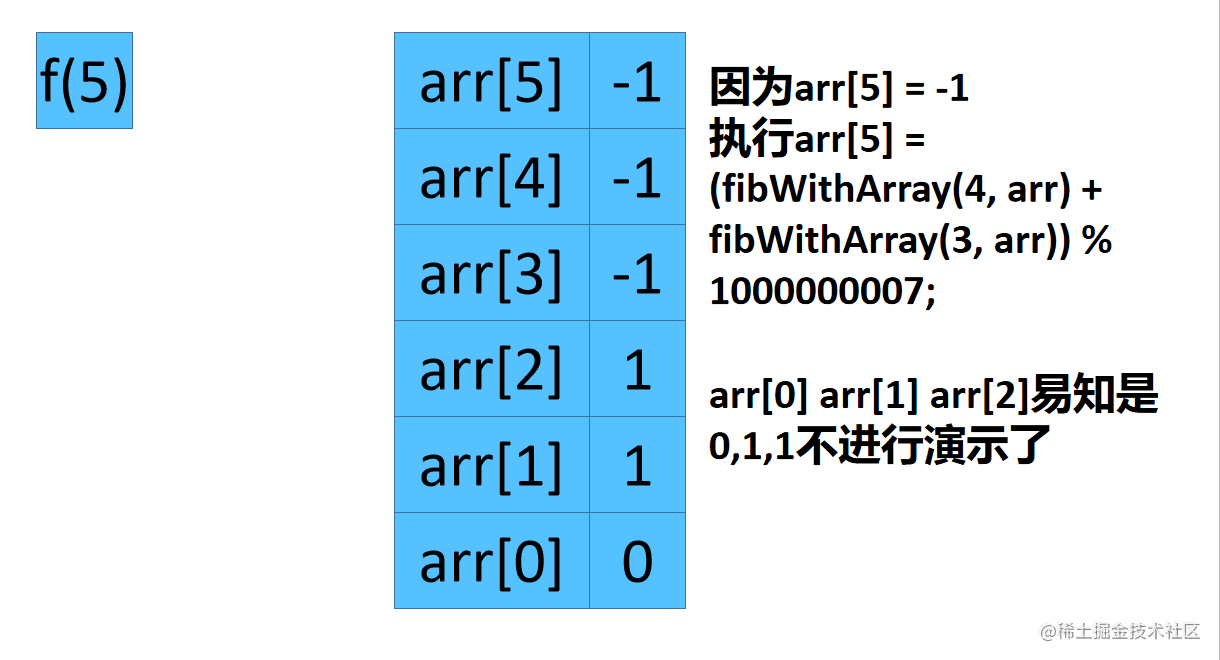### 动态规划解法动态规划的关键是要找出来转移方程，什么是状态转移方程？你把 `f(n)` 想做一个状态 `n`，这个状态 `n` 是由状态 `n - 1` 和状态` n - 2` 相加转移而来，这就叫状态转移。

``````class Solution {
public int fib(int n) {
if(n < 2)
return n;
int dp[] = new int[n + 1];
dp = 0;
dp = 1;
for(int i=2;i<=n;i++){
dp[i] = (dp[i-1] + dp[i-2]) % 1000000007;
}
return dp[n];
}
}

### 动态规划优化• 比如为了计算 dp, 需要先计算`dp = dp + dp`。 我们不使用数组，也就是`sum = b + a`。此时 `sum =1 b = 1 a= 0`
• 为了计算dp 需要保留dp也就是sum的计算结果。
• 于是我们让`a = b = 1` 也就是让a 保留 dp的结果 让`b = sum = 1`也就是让b保留dp的结果
• 所以dp = b + a = 2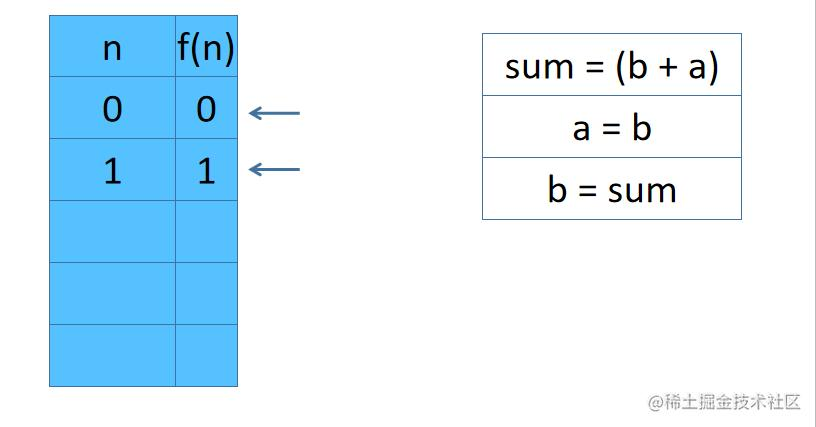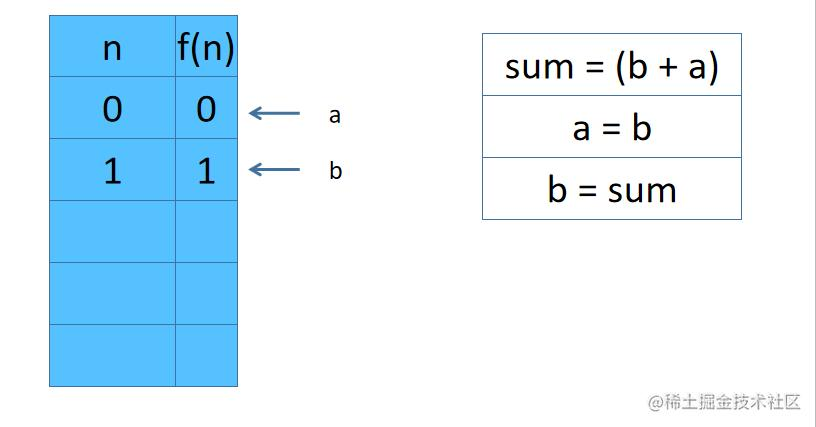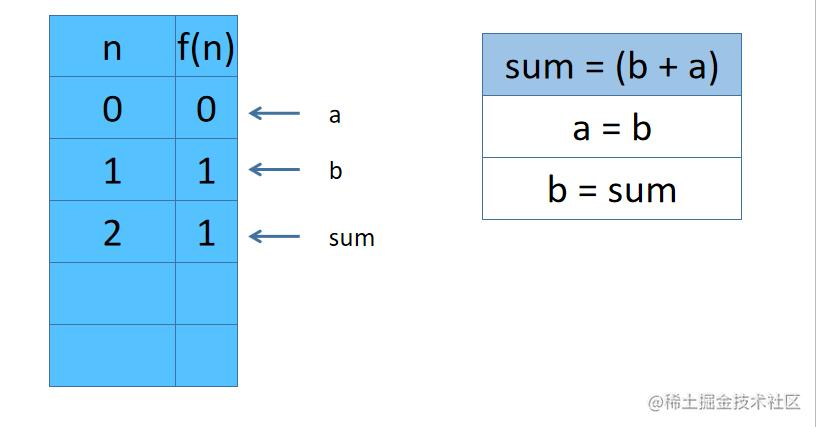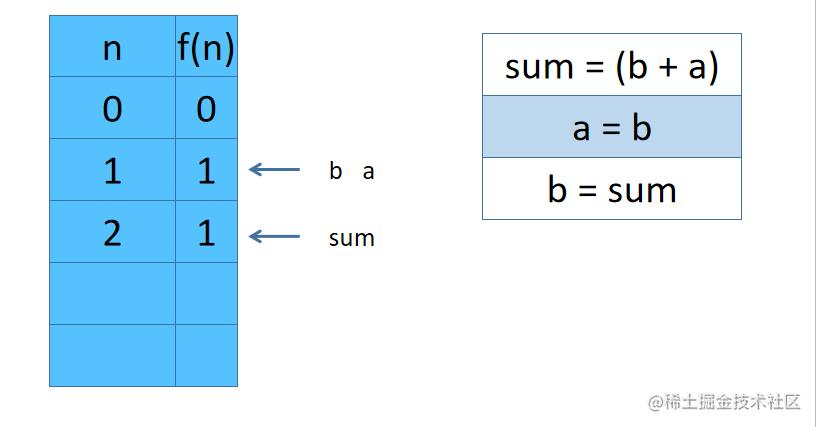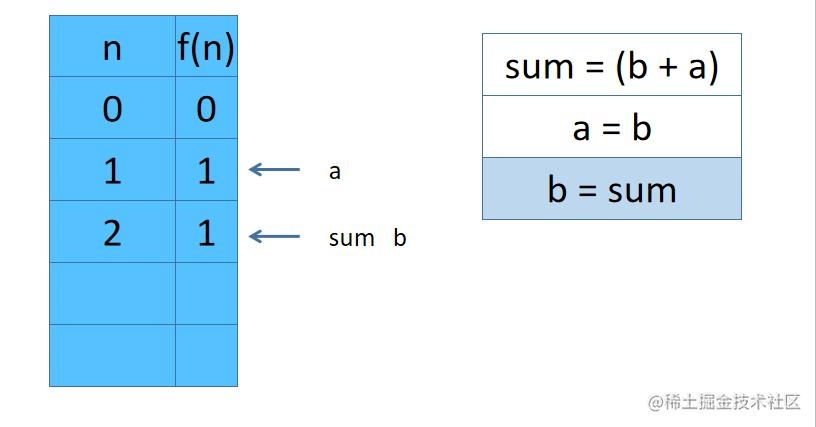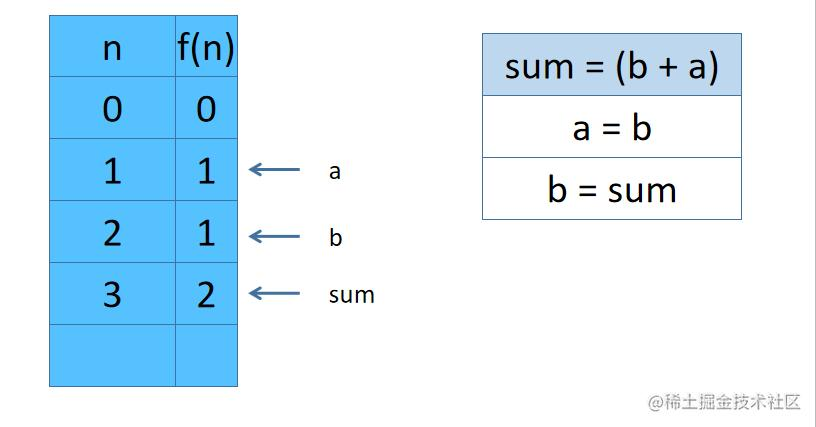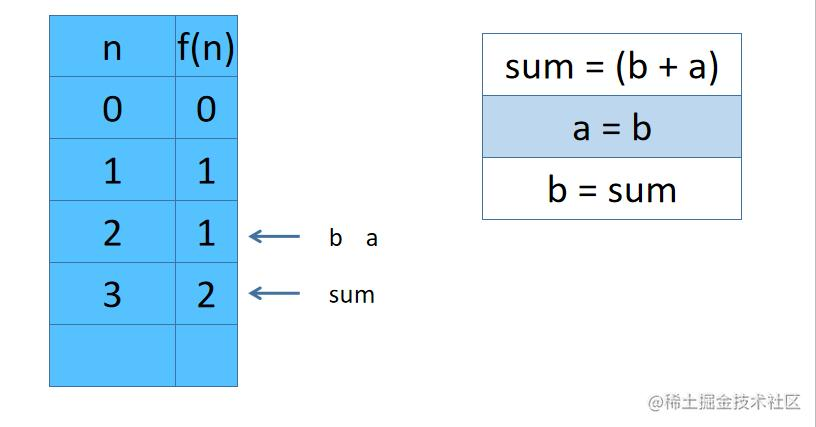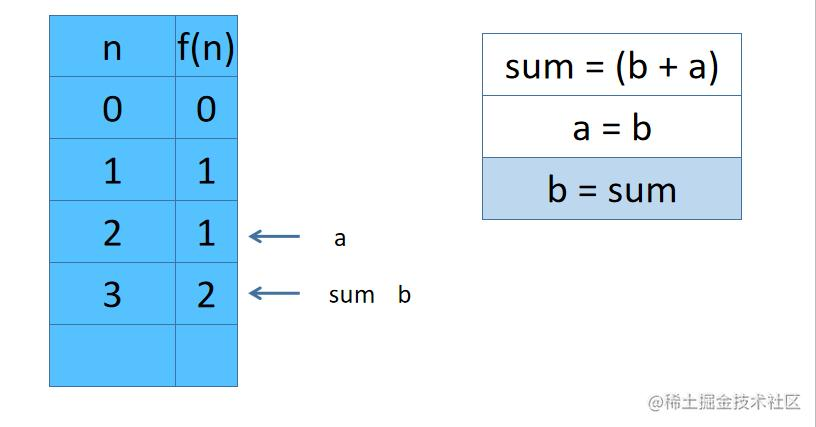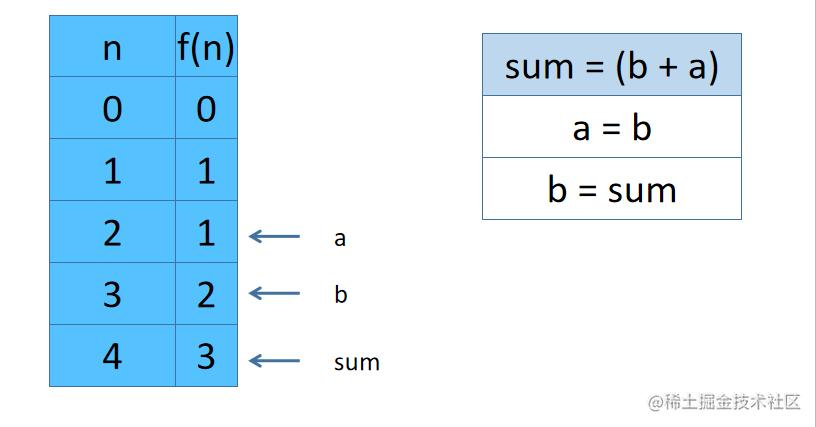### 动画演示一下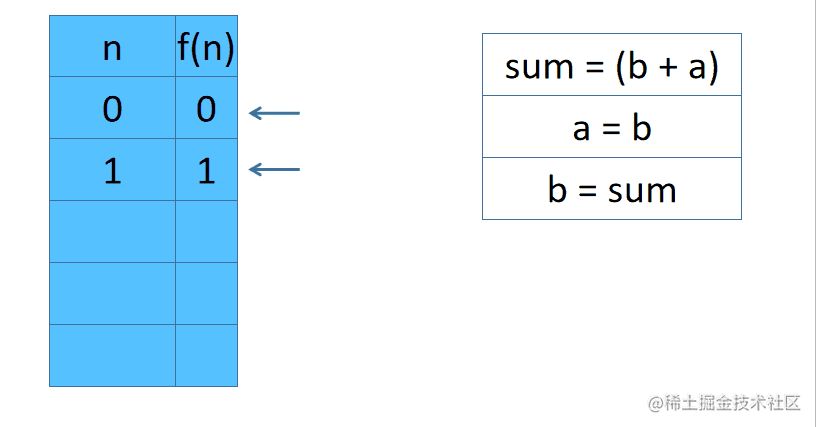### Java解法

``````class Solution {
public int fib(int n) {
int a = 0;
int b = 1;
if(n == 0)
return 0;
if(n == 1)
return 1;
int i = 2;
int sum=0;
while(i <= n)
{
sum = (a + b) % 1000000007;
a = b;
b = sum;
i++;
}
return sum;
}
}

### C++解法

``````class Solution {
public:
int fib(int n) {
int a = 0;
int b = 1;
if(n == 0)
return 0;
if(n == 1)
return 1;
int i = 2;
int sum=0;
while(i <= n)
{
sum = (a + b) % 1000000007;
a = b;
b = sum;
i++;
}
return sum;

}
};

### JS解法

``````/**
* @param {number} n
* @return {number}
*/
var fib = function(n) {
var a = 0;
var b = 1;
if(n == 0)
return 0;
if(n == 1)
return 1;
var i = 2;
var sum=0;
while(i <= n)
{
sum = (a + b) % 1000000007;
a = b % 1000000007;
b = sum;
i++;
}
return sum;
};

### PY解法

``````class Solution(object):
def fib(self, n):
a = 0;
b = 1;
if(n == 0):
return 0;
if(n == 1):
return 1;
i = 2;
sum=0;
while(i <= n):
sum = (a + b) % 1000000007;
a = b;
b = sum;
i += 1;
return sum;

### PHP解法

``````class Solution {

/**
* @param Integer \$n
* @return Integer
*/
function fib(\$n) {
\$a = 0;
\$b = 1;
if(\$n == 0)
return 0;
if(\$n == 1)
return 1;
\$i = 2;
\$sum=0;
while(\$i <= \$n)
{
\$sum = (\$a + \$b) % 1000000007;
\$a = \$b;
\$b = \$sum;
\$i++;
}
return \$sum;
}
}

### GO解法

``````func fib(N int) int {
a := 0;
b := 1;
if N == 0 {
return 0;
}
if N == 1 {
return 1;
}
sum := 0;
for i := 2; i <= N; i++ {
sum = (a + b) % 1000000007;
a = b;
b = sum;
}
return sum;
}

### 最后### 小夕最后有话说

• 动画、漫画、加6种编程语言（Java、C++、PHP、GO、Python、JS)的讲解很费心血，希望大家能帮忙转发到朋友圈和身边的朋友，这样小夕的文章能让更多的人看见的话，小夕会更有动力更新文章的呀~